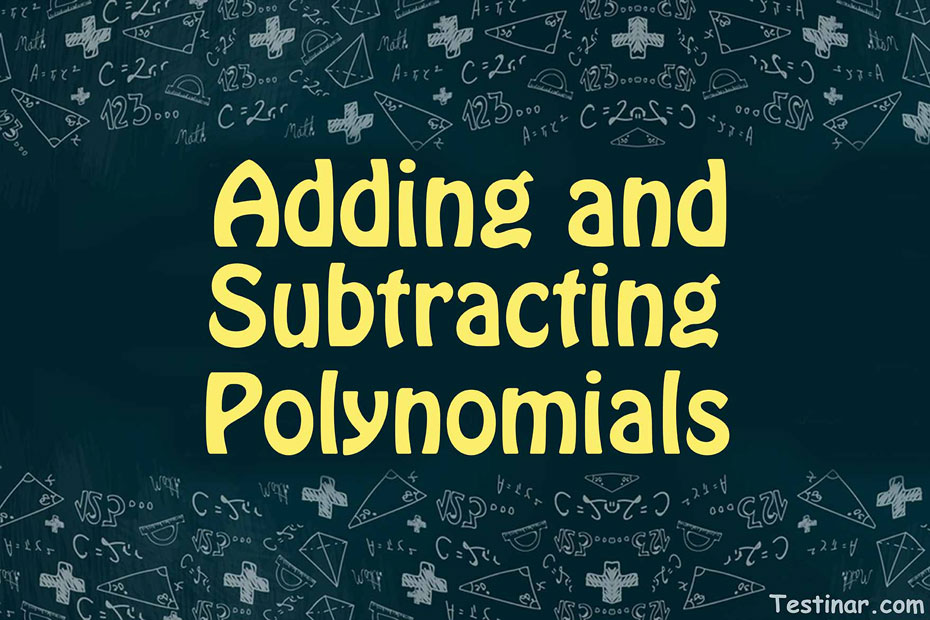## How to Add and Subtract Polynomials

The phrase "polynomial," as the name implies, is made up of the terms "poly" and "nomial," which relate to a variety of expressions. A polynomial is an algebraic or mathematical phrase consisting of at least two terms linked by a mathematical operation. Furthermore, the polynomial terms may include constants, variables, or a mixed term with a co-efficient. Furthermore, the variable's power (let's say $$x$$) in a polynomial should be an integer, not a fractional power like the squared or cube root.
For example, $$2x^{\frac{1}{3}} \ + \ 3x \ + \ 1$$ is not a polynomial as the first term has a fraction power of $$x$$.

### Types of Polynomials

Polynomials are classified into three categories based on the number of terms. Monomials, binomials, and trinomials are the three types. Let us go over these in greater detail:

• Monomial: They consist of just a single term but there is one condition. The term cannot be zero. Ex: 6x², 7x³, 67x, etc.
• Binomial: Binomials consist of just two terms and they must have a mathematical operation in between. Ex: 6x² + 7x³, 8x² + 7y, xy² + 3x, etc.
• Trinomial: Trinomial consist of three terms and between every two terms there is a mathematical operation. Ex: 2x² + 3x + 10, 4x³ + 3x² + 7, 3x²y + 4xy + 6, etc.

### Degree of a Polynomial Expression

To know the degree of a single variable expression, follow these steps:

• First, simplify the polynomial expression.
• Then, write the polynomial expression in the standard form.
• Next, you should check and select the highest exponent of the variable term.

Ex: $$4x^3 \ + \ 3x^2 \ + \ 7$$ has the degree $$3$$ since the highest exponent of $$x$$ is $$3$$.

To know the degree of a multivariable polynomial expression, follow these steps:

• First, simplify the polynomial expression.
• Then, write the polynomial expression in the standard form.
• Next, you should check and select the highest exponent of the variable term. Note: you should add the powers of different variables too.

Ex: $$x^3 \ + \ 3x^2y^3 \ + \ 4$$ has the degree $$5$$, since the middle term has the power of $$x \ = \ 2$$ and $$y \ = \ 3$$, so the addition comes to $$5$$.

### Addition and Subtraction in Polynomials

To do addition or subtraction in a polynomial expression, apply the below-mentioned steps:

• First, simplify the expression by adding/subtracting the like terms. Also. Don’t forget to apply PEMDAS rule in case of brackets or parenthesis.
• Also, wherever possible, use the distributive property.

Ex: $$(3x^3 \ - \ 2) \ – \ (2x^3 \ + \ 4) \ = \ 3x^3 \ - \ 2x^3 \ - \ 2 \ – \ 4 \ = \ x^3 \ - \ 6$$.

### Exercises for Adding and Subtracting Polynomials

1) $$(-9x \ + \ 8) \ + \ (6x \ - \ 6) =$$

2) $$(6x \ + \ 3) \ + \ (2x \ - \ 2) =$$

3) $$(-7x \ + \ 9) \ - \ (6x \ + \ 7) =$$

4) $$(2x^2 \ + \ 7x) \ - \ (2x^2 \ + \ 3x) =$$

5) $$(8x \ + \ 9) \ - \ (6x \ + \ 7) =$$

6) $$(-5x^2 \ + \ 6x) \ - \ (4x^2 \ + \ 4x) =$$

7) $$(4x^2 \ + \ 7x) \ + \ (4x^2 \ - \ 5x) =$$

8) $$(-3x^2 \ + \ 5x) \ + \ (5x^2 \ - \ 3x) =$$

9) $$(-4x^2 \ + \ 7x) \ - \ (4x^2 \ + \ 5x) =$$

10) $$(10x^2 \ + \ 5x) \ - \ (5x^2 \ + \ 4x) =$$

1) $$(-9x \ + \ 8) \ + \ (6x \ - \ 6) =$$$$\ \color{red}{-3x \ + \ 2}$$
2) $$(6x \ + \ 3) \ + \ (2x \ - \ 2) =$$$$\ \color{red}{8x \ + \ 1}$$
3) $$(-7x \ + \ 9) \ - \ (6x \ + \ 7) =$$$$\ \color{red}{-13x \ + \ 2}$$
4) $$(2x^2 \ + \ 7x) \ - \ (2x^2 \ + \ 3x) =$$$$\ \color{red}{0x^2 \ + \ 4x}$$
5) $$(8x \ + \ 9) \ - \ (6x \ + \ 7) =$$$$\ \color{red}{2x \ + \ 2}$$
6) $$(-5x^2 \ + \ 6x) \ - \ (4x^2 \ + \ 4x) =$$$$\ \color{red}{-9x^2 \ + \ 2x}$$
7) $$(4x^2 \ + \ 7x) \ + \ (4x^2 \ - \ 5x) =$$$$\ \color{red}{8x^2 \ + \ 2x}$$
8) $$(-3x^2 \ + \ 5x) \ + \ (5x^2 \ - \ 3x) =$$$$\ \color{red}{2x^2 \ + \ 2x}$$
9) $$(-4x^2 \ + \ 7x) \ - \ (4x^2 \ + \ 5x) =$$$$\ \color{red}{-8x^2 \ + \ 2x}$$
10) $$(10x^2 \ + \ 5x) \ - \ (5x^2 \ + \ 4x) =$$$$\ \color{red}{5x^2 \ + \ 1x}$$

## Adding and Subtracting Polynomials Practice Quiz

### HiSET Mathematics Formulas

$6.99$5.99

### TASC Math Study Guide 2020 – 2021

$20.99$15.99

### Accuplacer Math for Beginners

$24.99$13.99

### The Most Comprehensive GED Math Preparation Bundle

$79.99$34.99